• 概率学上，对未知概率密度函数进行估计有两种方法参数估计和非参数估计。非参数估计是不假定数学模型，直接利用已知类别学习样本先验知识估计数学模型。而参数估计则是先假定研究问题具有某种数学模型，如正态...

所谓估计

概率学上，对未知的概率密度函数进行估计有两种方法：参数估计和非参数估计。非参数估计是不假定数学模型，直接利用已知类别的学习样本先验知识估计数学模型。常用的方法由直方图方法、神经网络方法、Parzen窗法和Kn$K_n$近邻法。而参数估计则是先假定研究问题具有某种数学模型，如正态分布、二项分布等，再利用已知类别的学习样本，估计模型里的参数。常用的方法有距估计、最大似然估计、最大后验估计和贝叶斯估计。本文主要介绍四种常用的参数估计技术。

参数估计

1. 距估计
用样本矩作为相应总体矩的估计量，而以样本矩的连续函数作为相应的总体矩的连续函数的估计量。用数学公式描述矩估计的过程为：
⎧⎩⎨⎪⎪⎪⎪⎪⎪⎪⎪μ1=μ1(θ1,θ2,...,θk)μ2=μ2(θ1,θ2,...,θk)......μk=μk(θ1,θ2,...,θk)⎫⎭⎬⎪⎪⎪⎪⎪⎪⎪⎪
\left\{
\begin{matrix}
\mu_1=\mu_1(\theta_1,\theta_2,...,\theta_k)  \\
\mu_2=\mu_2(\theta_1,\theta_2,...,\theta_k)   \\
... ...\\
\mu_k=\mu_k(\theta_1,\theta_2,...,\theta_k)
\end{matrix}
\right\}

从中解出参数
⎧⎩⎨⎪⎪⎪⎪⎪⎪⎪⎪θ1=θ1(μ1,μ2,...,μk)θ2=θ2(μ1,μ2,...,μk)......θk=θk(μ1,μ2,...,μk)⎫⎭⎬⎪⎪⎪⎪⎪⎪⎪⎪
\left\{
\begin{matrix}
\theta_1=\theta_1(\mu_1,\mu_2,...,\mu_k)  \\
\theta_2=\theta_2(\mu_1,\mu_2,...,\mu_k)   \\
... ...\\
\theta_k=\theta_k(\mu_1,\mu_2,...,\mu_k)
\end{matrix}
\right\}

其中，θ1,θ2,...,θk$\theta_1,\theta_2,...,\theta_k$是k个待估参数， μ1,μ2,...,μk$\mu_1,\mu_2,...,\mu_k$是总体k阶矩。先用已知样本，计算k阶样本矩，公式为：
Al=∑Ni=1XliN
A_l=\frac {\sum_{i=1}^NX_i^l} {N}

然后用计算得到的k阶样本矩来作为对总体矩的估计，带入方程得到对应的矩估计：
θ¯l=θi(A1,A2,...,Ak)
\overline\theta_l=\theta_i(A_1,A_2,...,A_k)

2. 最大似然估计（MLE）
样本X1,X2,...,Xn$X_1,X_2,...,X_n$来自总体X，总体的概率密度为P{X=x}=p(x;θ)$P\{X=x\}=p(x;\theta)$或f(x;θ)$f(x;\theta)$。其中θ∈Θ$\theta\in\Theta$的形式已知，θ$\theta$为待估参数。得到其似然函数为：
L(θ)=L(x1,x2,...,xn;θ)=∏i=1nf(xi;θ)
L(\theta)=L(x_1,x_2,...,x_n;\theta)=\prod_{i=1}^nf(x_i;\theta)

那么，当L(x1,x2,...,xn;θ)$L(x_1,x_2,...,x_n;\theta)$在θ∈Θ$\theta\in\Theta$中取得最大值时，即公式描述为：
L(x1,x2,...,xn;θ¯)=maxθ∈ΘL(x1,x2,...,xn;θ)
L(x_1,x_2,...,x_n;\overline\theta)=max_{\theta\in\Theta} L(x_1,x_2,...,x_n;\theta)

θ¯$\overline \theta$就是θ$\theta$的最大似然估计θ¯(x1,x2,...,xn)$\overline\theta(x_1,x_2,...,x_n)$。在应用中常常采用对数形式给出对数似然方程，在计算中，令dL(θ)dθ=0$\frac {dL(\theta)}{d\theta}=0$或者dlogL(θ)dθ=0$\frac {d\log L(\theta)}{d\theta}=0$，得到最大值处的θ$\theta$就是最大似然估计。

3. 最大后验估计（MAP）
最大似然估计没有考虑θ$\theta$的概率分布，或者认为θ$\theta$的概率分布在θ∈Θ$\theta\in\Theta$上式均匀分布的。在贝叶斯学派看来，θ$\theta$也是随机变量，有着一定的先验概率。因此如果不加以考虑，估计结果会出现较大的误差。最大后验估计的表达式为：
p(θ|x1,x2,...,xn)=p(x1,x2,...,xn|θ)×p(θ)∑i{p(x1,x2,...,xn|θi)×p(θi)}=L(x1,x2,...,xn|θ)×p(θ)const
p(\theta|x_1,x_2,...,x_n)=\frac {p(x_1,x_2,...,x_n|\theta) \times p(\theta)} {\sum_i\{p(x_1,x_2,...,x_n|\theta_i)\times p(\theta_i)\}}=\frac{L(x_1,x_2,...,x_n|\theta)\times p(\theta)}{const}

公式可以等效为：
后验概率=(似然度×先验概率)标准化常量=标准似然度×先验概率
后验概率=\frac{(似然度\times 先验概率)}{标准化常量}=标准似然度\times 先验概率

4. 贝叶斯估计
贝叶斯估计也是基于后验概率公式，但引入了损失函数作为判断的标准。贝叶斯估计得一般步骤为

选择先验概率分布，设为π(θ)$\pi(\theta)$
确定似然函数。
确定参数θ$\theta$的后验分布。
选择损失函数。
引入一个非负函数，记为loss(θ^,θ)$loss(\hat\theta,\theta)$来刻画参数真实值θ$\theta$与估计值θ^$\hat\theta$的差距严重程度，称为损失函数。常用的损失函数有：平方误差损失函数
估计参数。
根据选择的损失函数的期望误差最小值对应的解θ^$\hat\theta$作为参数的贝叶斯估计值。以平方误差损失函数为例，贝叶斯估计给定X时的条件期望为：
θ^=E[θ|X]=∫θp(θ|X)dθ
\hat\theta=E[\theta|X]=\int\theta p(\theta|X)d\theta

2015-8-22
艺少
展开全文密度估计 统计学 似然函数
• 在机器学习中，监督式学习（Supervised Learning）通过定义一个模型，并根据训练集上数据估计最优参数。梯度下降法（Gradient Descent）是一个广泛被用来最小化模型误差的参数优化算法。梯度下降法通过多次迭代，...
学习率 Learning Rate本文从梯度学习算法的角度中看学习率对于学习算法性能的影响，以及介绍如何调整学习率的一般经验和技巧。在机器学习中，监督式学习（Supervised Learning）通过定义一个模型，并根据训练集上的数据估计最优参数。梯度下降法（Gradient Descent）是一个广泛被用来最小化模型误差的参数优化算法。梯度下降法通过多次迭代，并在每一步中最小化成本函数（cost function）来估计模型的参数（weights）。 梯度下降的伪代码如下： 　　重复已下过程，直到收敛为止{　　　　ωj = ωj - λ ∂F(ωj) / ∂ωj　　} 说明：（1） ωj 是模型参数， F()是成本函数， ∂F(ωj) / ∂ωj 是ωj  的一阶导数，λ 是学习率　　　（2）如果F()是单调函数，经过多次迭代会得到最小的成本函数；如果F()非单调，那么我们有可能陷入局部最优，一个简单的解决办法是通过多次尝试不同的ωj 初始值，对比不同估计参数下的成本函数的值是否一致，来发现是否陷入局部最优。　　　（3）梯度下降法未必是最优的计算权重参数的方法，但是作为一种简单快速的方法，常常被使用。参照Andrew Ng的Stanford公开课程。  梯度下降过程的图示如下：学习率的调整为了能够使得梯度下降法有较好的性能，我们需要把学习率的值设定在合适的范围内。学习率决定了参数移动到最优值的速度快慢。如果学习率过大，很可能会越过最优值；反而如果学习率过小，优化的效率可能过低，长时间算法无法收敛。所以学习率对于算法性能的表现至关重要。 对于不同大小的数据集，调节不同的学习率根据我们选择的成本函数F()不同，问题会有区别。当平方误差和（Sum of Squared Errors）作为成本函数时， ∂F(ωj) / ∂ωj 会随着训练集数据的增多变得越来越大，因此学习率需要被设定在相应更小的值上。解决此类问题的一个方法是将学习率λ 乘上1/N，N是训练集中数据量。这样每部更新的公式变成下面的形式：ωj = ωj - (λ/N) * ∂F(ωj) / ∂ωj相关内容可参考： Wilson et al. paper “The general inefﬁciency of batch training for gradient descent learning”另外一种解决方法是：选择一个不被训练集样本个数影响的成本函数，如均值平方差（Mean Squared Errors）。 在每次迭代中调节不同的学习率在每次迭代中去调整学习率的值是另一种很好的学习率自适应方法。此类方法的基本思路是当你离最优值越远，你需要朝最优值移动的就越多，即学习率就应该越大；反之亦反。但是这里有一个问题，就是我们并不知道实际上的最优值在哪里，我们也不知道每一步迭代中我们离最优值有多远。 解决办法是，我们在每次迭代的最后，使用估计的模型参数检查误差函数（error function）的值。如果相对于上一次迭代，错误率减少了，就可以增大学习率，以5%的幅度；如果相对于上一次迭代，错误率增大了（意味着跳过了最优值），那么应该重新设置上一轮迭代ωj 的值，并且减少学习率到之前的50%。这种方法叫做 Bold Driver. 建议：归一化输入向量归一化输入向量在机器学习问题中是一个通用的方法。在一些应用中，由于使用距离或者特征方差，要求必须归一化输入向量，因为如果不归一化将导致结果会严重被具有大方差的特征和不同的尺度影响。归一化输入能够帮助数值最优方法（例如，梯度下降法）更快，更准确地收敛。尽管有一些不同的归一化变量的方法，[0,1]归一化（也叫做min-max）和z-score归一化是两种最为广泛应用的。 XminmaxNorm = (X - min(X)) / (max(X) - min(X));XzscoreNorm = (X - mean(X)) / std(X);（第一个是非标准的归一化，第二个是正太化），两个可以做一个二选一，进行数据标准化！！！！第一个的范围是0-1第二个的范围是无穷，概率积分后落入-3δ---3δ第二个就是把数据编程了均值为0，方差为1 的一组数，只是把数据位移了一下， 然后在Y轴上压扁了一下，但范围还是无穷
展开全文• 文章目录1、前言2、最大似然估计法 MLE3、最大后验估计 MAP4...在概率论中，参数估计有点估计(point estimation)和区间估计(interval estimation)两种。而 ML 中主要是构造点估计的方法常用的有：①最大似然估计法，...
文章目录1、前言2、最大似然估计法 MLE3、最大后验估计 MAP4、贝叶斯估计5、其他的参数估计方法
1、前言
我们讨论的是有参的情况，在这种情况中，我们的目标是估计参数值（假设有可能确定真是参数），而不是函数值。在概率论中，参数估计有点估计(point estimation)和区间估计(interval estimation)两种。而 ML 中主要是构造点估计的方法常用的有：①最大似然估计法，用来求一个样本集的相关概率密度函数的参数；②最小二乘法，主要用于线性统计模型中的参数估计问题；③贝叶斯估计法；等等。
2、最大似然估计法 MLE
MLE（maximum likelihood estimation）通常是机器学习中首选的估计方法，因为它具有一致性（当训练样本数目趋向无穷大时，参数的最大似然估计就会收敛到参数的真实值） 和 统计效率（输入与输出存在不平衡的关系）。
最大似然参数求解的核心思想就是构造当前样本出现的联合概率函数，对其求偏导，让当前样本的概率最大的就是模型参数。
考虑一组含有 m 个样本的数据集X=$x_1,x_2,...,x_m\brace$ ，独立地由未知的真实数据生成分别$p_{data} (x)$生成。令 $p_{model} (x;\theta)$是一族由$\theta$确定的相同空间上的概率分布。对$\theta$的MLE被定义为：
$\theta_{ML} = \arg\max_{\theta}p_{model} (X;\theta) =\arg\max_{\theta}\prod_{i}^{m} p(x_i;\theta) \tag{1}$求解过程：通常将乘积转成求和（乘积会导致在计算中出现数值下溢），也就是取对数，然后再进行求偏导等操作。
$\theta_{ML} = \arg\max_{\theta} \sum_{i}^{m} \log p(x_i;\theta) \tag{2}$
和MLE类似的参数估计方法还有：

条件对数似然：估计条件概率$p(y|x;\theta)$，从给定x预测y:$\theta_{ML} = \arg\max_{\theta} \sum_{i}^{m} \log p(y_i|x_i;\theta) \tag{3}$
KL散度

3、最大后验估计 MAP
MAP 估计选择后验概率最大的点（或在$\theta$是连续纸的更常见的情况下，概率密度最大的点）为最终的模型参数。$\theta_{MAP} = \arg\max_{\theta}p(\theta|x)= \arg\max_{\theta}\log p(x|\theta) +\log p(\theta) \tag{4}$可以看出右项中$\log p(x|\theta)$对应这标准的对数似然，$\log p(\theta)$对应着先验分布。

MAP增加了先验信息，有助于减少最大后验估计的方差，但是也增加了偏差；
具有高斯先验权重的MAP贝叶斯推断对应着权重衰减，此时先验项$\log p(\theta)$正比于权重衰减惩罚$\lambda w^Tw$ (MAP提供了一个直观的方法来设计复杂但可解释的正则化项)；权重衰减正则化的最大似然学习，就可以解释为贝叶斯推断的MAP近似。
MLE和MAP都是估计单一值$\theta$的方法，但是MLE是频率派统计方法，而MAP是贝叶斯派方法。

4、贝叶斯估计
贝叶斯估计是在MAP上做进一步拓展，此时不直接估计参数的值，而是允许参数服从一定概率分布（MLE和MAP都是估计单一值$\theta$的方法）。
贝叶斯估计通过贝叶斯规则结合数据似然和先验，可以恢复数据对我们关于$\theta$的信念的影响：$p(\theta|x_1,x_2,...,x_m)=\frac{p(x_1,x_2,...,x_m|\theta)p(\theta)}{p(x_1,x_2,...,x_m)}$
预测：$p(x_{m+1}|x_1,x_2,...,x_m)=\int{p(x_{m+1}|\theta)p(\theta|x_1,x_2,...,x_m)}d_{\theta}$

预测时，贝叶斯估计使用的$\theta$的全分布
当训练数据有限时，贝叶斯方法通常泛化得更好，当数据量很大时，通常会有很大的计算代价。

5、其他的参数估计方法

求解算法的模型参数的常用方法
适用范围
过程
优点
缺点
其他

OLS
用来做函数拟合或者求函数极值的方法（在机器学习，尤其是回归模型中，常用）
1、 线性：$\red{\theta=(X^TX)^{-1}X^TY}$2、 非线性：迭代法
适用简洁高，  不需要选择步长， 也不用迭代求解，  最小二乘法是计算解析解。
$1. \red{X^TX 要可逆}$，            2. 如果样本量很大，用最小二乘法由于需要求一个超级大的逆矩阵，这时就很难或者很慢才能求解解析解了，使用迭代的梯度下降法比较有优势。                                     3. 拟合函数需要是线性的

带权的最小二乘（WLS，IRLS）
用于线性回归、逻辑回归、AFT生存回归和多层感知器的求解
1、线性化目前的解决方案的目标，并更新相应的权重。2、通过Weighted Least Squares解决加权最小二乘（WLS）问题。3、重复上述步骤直到收敛。
加入了权重矩阵，进一步提高状态估计精度
为了使正则方程逼近是有效的，加权最小二乘要求特征的数量不超过4096个。  对于规模更大的问题，是有L-BFGS。

Gradient Descent  （BGD，SGD，MBGD
在最小化损失函数时，可以通过梯度下降法来一步步的迭代求解，得到最小化的损失函数，和模型参数值。
1、根据miniBatch Fraction参数进行样本抽样，获得一个小样本集;    2、调用Gradient计算在小样本集上的梯度值；  3、调用Updater,根据regParam、stepSize、numIterations等参数值更新模型参数；  4、判断终止条件(精度收敛或者迭代次数达到上限)，否则继续上面步骤。
迭代求解，速度较快
需选择步长和初值；  局部最优解（梯度下降可以逼近解析解，要保证你的训练数据是凸）
梯度下降法和最小二乘法相比：  梯度下降法需要选择步长，而最小二乘法不需要。  梯度下降法是迭代求解，最小二乘法是计算解析解。  如果样本量不算很大，且存在解析解，最小二乘法比起梯度下降法要有优势，计算速度很快。  但是如果样本量很大，用最小二乘法由于需要求一个超级大的逆矩阵，这时就很难或者很慢才能求解解析解了，使用迭代的梯度下降法比较有优势。

牛顿法
非线性逼近
解方程组→得到搜索方向→移动点→检验收敛条件
收敛快，二阶收敛，因此它比最速下降法要快
需要计算嗨森矩阵，对一般问题不是整体收敛的，只有当初始点充分接近极小点时，才有很好的收敛性，  牛顿法的鲁棒性较差（H要正定）
梯度下降法和牛顿法/拟牛顿法相比:  两者都是迭代求解，不过梯度下降法是梯度求解，而牛顿法/拟牛顿法是用二阶的海森矩阵的逆矩阵或伪逆矩阵求解。  相对而言，使用牛顿法/拟牛顿法收敛更快。但是每次迭代的时间比梯度下降法长。

拟牛顿法（lbfgs
非线性逼近
算法只保存并利用最近m次迭代的曲率信息来构造海森矩阵的近似矩阵。
收敛速度介于梯度下降法和牛顿法之间，是超线性的。  L-BFGS方法以二次方程来逼近目标函数来构造黑塞矩阵，不考虑目标函数的二阶偏导数。  L-BFGS通常比其他一阶优化方法能更快收敛。每次迭代的开销非常小，每一步迭代都能保证近似矩阵的正定，因此算法的鲁棒性还是很强的。


展开全文机器学习
• 参数估计分点估计和区间估计两种。 点估计 点估计是用样本统计量确定总体参数的一个数值。 评价估计优劣的标准有无偏性、 小方差性、有效性等，估计的方法有矩法、极大似然法等。 常用的是对总体均值 μ 和...
利用样本对总体进行统计推断的一类问题是参数估计，即假定已知总体的分布，通 常是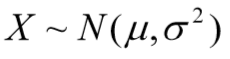，估计有关的参数，如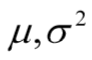。
参数估计分点估计和区间估计两种。

点估计

点估计是用样本统计量确定总体参数的一个数值。
评价估计优劣的标准有无偏性、 小方差性、有效性等，估计的方法有矩法、极大似然法等。
常用的是对总体均值 μ 和方差 σ2 （或标准差 σ ）作点估计。当从一个样本按照式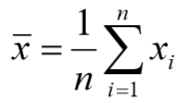、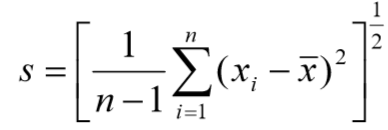算出样本均值 x 和方差 s 2 后，对μ 和 σ2 （或σ ）一个自然、合理的点估计显然是（在字母上加^表示它的估计值）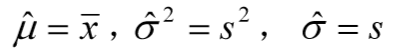区间估计

点估计虽然给出了待估参数的一个数值，却没有告诉我们这个估计值的精度和可信 程度。
一般地，总体的待估参数记作 θ （如 μ  , σ2），由样本算出的 θ 的估计量记作 θ ˆ ， 人们常希望给出一个区间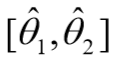使得θ 以一定的概率落在此区间内。若有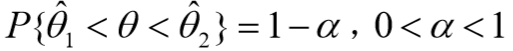则称为 θ 的置信区间，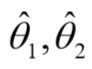分别称为置信下限和置信上限，
α −1 称为置信概率或置信水平， α 称为显著性水平。

给出的置信水平为α −1 的置信区间，称为 θ 的区间估计。置信区间越小， 估计的精度越高；置信水平越大，估计的可信程度越高。
但是这两个指标显然是矛盾的， 通常是在一定的置信水平下使置信区间尽量小。
通俗地说，区间估计给出了点估计的误 差范围。

转载于:https://www.cnblogs.com/wander-clouds/p/9060495.html
展开全文• 在有限的样本下，如何判定哪个估计最优，概率论中有两种常用的principle：MLE（Maximum likelihood estimation），MAP(Maximum a posteriori estimation)。由于估计的是一个确定的参数值，MLE和MAP称为点估计。事实...
• 参数估计就是根据样本统计量的数值对总体参数进行估计的过程。根据参数估计的性质不同，可以分成两种类型：点估计和区间估计。 点估计 点估计就是用样本统计量的某一具体...对总体参数进行点估计常用的方法两种...统计学
• 常用的模式分类的非参数技术主要有两种：Parzen窗估计方法和K-近邻概率密度估计方法。二者其实是对同一个问题的不同角度去解决。Parzen窗估计方法的主题思想是固定窗口区域容积，去看有多少个样本点在里面，而K-近邻...机器学习 模式分类 MATLAB
• 参数估计两种形式：点估计与区间估计。本文选择几种常用的点估计方法作一些讨论。 用于估计未知参数的统计量称为点估计（量）。参数 θθ\theta 的估计量常用 θ^=θ^(x1,x2,…,xn)θ^=θ^(x1,x2,…,xn)\hat{\...数理统计
• 本文原始地址：最大似然估计（Maximum likelihood estimation, 简称MLE）和最大后验概率估计（Maximum a posteriori estimation, 简称MAP）是很常用的两种参数估计方法，如果不理解这两种方法的思路，很机器学习 贝叶斯
• 常用的参数估计方法有直方图法和核密度估计方法。 常采用高斯核，带宽h（平滑参数）通常采用交叉验证得到最优值。 MATLAB实现 参考https://www.mathworks.com/help/stats/ksdensity.html % The estimate...
• 参数估计（笔记一）统计推断问题可以分为两类：1、估计...下面介绍两种常用的构造估计量的方法：矩估计和最大似然法。 矩估计 由上面的定义可知，基于总体X的k阶矩是待估参数的函数且一定存在。基于样本的k阶矩数学变量
• 下能对常用的11数字调制信号CW、BPSK、QPSK、OQPSK、16QAM，32QAM， 64QAM、2FSK、4FSK、GMSK和OFDM进行准确的识别。 其次，研究了非协作通信中观测信号调制参数估计问题。对M-PSK及 M-QAM信号滚降系数的研究...
• 参数估计问题是统计学中非常经典问题，对于此类问题，我们将主要讨论两种常用和很有效的方法,也就是:最大似然估计和贝叶斯估计。 最大似然估计(MLE)与最大后验概率估计(MAP)非常相似，具体内容可查看：【模式...机器学习 人工智能 数据分析
• 【机器学习基本理论】详解最大后...最大似然估计（Maximum likelihood estimation, 简称MLE）和最大后验概率估计（Maximum a posteriori estimation, 简称MAP）是很常用的两种参数估计方法，如果不理解这两种方法的...MAP
• 最大似然估计（Maximum likelihood estimation, 简称MLE）和最大后验概率估计（Maximum a posteriori estimation, 简称MAP）是很常用的两种参数估计方法，如果不理解这两种方法的思路，很容易弄混它们。下文将详细...
• 现代功率谱估计和经典功率谱估计两种常用的功率谱估计，同时也是分析随机信号的常用方法。本文详细介绍了现代功率谱估计中有关AR模型参数的功率谱估计，具体包括自相关算法、Burg算法、协方差算法以及改进的协方差...
• 最大似然估计（Maximum likelihood estimation, 简称MLE）和最大后验概率估计（Maximum a posteriori estimation, 简称MAP）是很常用的两种参数估计方法，如果不理解这两种方法的思路，很容易弄混它们。下文将详细说...
• 两种方法的生存概率估计比较显示，与Kaplan-Meier相比，参数化方法生存概率估计值更好。 参数生存分析更加健壮和高效，因为它基于定义明确的参数概率分布，因此优于非参数Kaplan-Meier。 这项研究为进一步...
• 摘要最大似然估计(Maximum Likelihood Estimation)与最大后验估计(Maximum A Posteriori)是机器学习中最常用的两种点估计参数估计方法. 最大似然估计以最大化观测数据集上的似然度为目标, 强调从观测数据集上拟合出...
• 最大似然估计（Maximum likelihood estimation, 简称MLE）和最大后验概率估计（Maximum a posteriori estimation, 简称MAP）是很常用的两种参数估计方法，如果不理解这两种方法的思路，很容易弄混...
• 极大似然估计（Maximum Likelihood Estimation，MLE）和贝叶斯估计（Bayesian Estimation）是统计推断中两种常用的参数估计方法，二者在机器学习中的应用也十分广泛。本文将对这两种估计方法做一个详解。考虑这样......